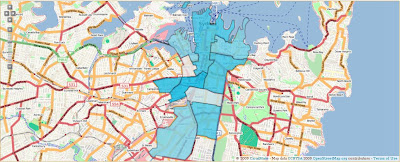# Generating KML Thematic MapsThe Biarri workbench now has a tool for showing the frequency of an event per postcode. The colourised map (funkier name Choropleth map) is generated as KML from postcode regions that are stored in a postgis database. This just requires a little bit of sql and python:

``````
dict_cur.execute("select p.gid, p.poa_2006, ST_AsKML(the_geom) as \
polygon, frequency, (frequency - stats.min) / (stats.max - \
stats.min)::float as scaled_frequency from "+table+" c, \
postcode_regions p, (select max(frequency), min(frequency) \
from "+table+") as stats where p.poa_2006 = c.postcode")
return my_lookup.get_template("thematic_kml.mako").render(rows=dict_cur.fetchall())
``````

The sql just normalises the column we want as our colour intensity and returns the KML for each postcode region.

The mako template:

``````

Thematic Map
generated with the Biarri workbench
% for row in rows:

\${row['poa_2006']}

\${row['frequency']}

#\${row['gid']}
\${row['polygon'].replace(" ", '\n')}

% endfor

<%!
import webcolors
import colorsys

def intensity(frequency):
#frequencies are already scaled between 0 and 1
return 'cc' webcolors.rgb_to_hex(tuple((int(a * 256) for a in colorsys.hsv_to_rgb(0.134, frequency, 0.90)))).strip('#')
%>
``````

The mako template is quite easy to read if you notice the % sections are actually python code. The only challenge I had was that ST_AsKML produces kml with the co-ordinates space separated. Google earth is fine with this but the kml viewer we’re using, web map lite, only likes them newline separated. My colour function just changes the intensity of the colour. A gradient between blue and red would probably be more appropriate.

Loki

Tags:
0 replies